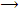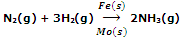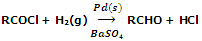Explain the catalyst definition and process with examples.

Catalyst is a substance which accelerates the rate of a chemical reaction without undergoing any change in its chemical composition or mass during the reaction. The phenomenon of increasing the rate of a reaction with the help of a catalyst is known as catalysis.

For example, decomposition of potassium chlorate to give dioxygen occurs at high temperature in the range of 653 - 873 K

2KClO32KCl + 3O2

However, if a small amount of MnO2 is added to KClO3, its decomposition becomes faster and occurs at lower temperature range 473 - 633 K. the mass and chemical composition of MnO2 remains unaltered at the end of reaction. Thus, MnO2 acts as catalyst for the decomposition of KClO3.

Catalytic action

Since the catalysts are not consumed during the reaction, therefore, only a small amount of catalyst is sufficient to catalyse the reaction. According to modern views, a catalyst speeds up the reaction by providing an alternate path of lower activation energy to the reactants. The catalyst lowers the activation energy by interacting with the reactants leading to the formation of some intermediate complex of lower potential energy. In due course, the intermediate complex decomposes to give the products and also the catalyst.

Promotors and poisons

Promotors are the substances which enhance the activity of catalysts. For example, in the Hber's process for the manufacture of ammonia, molybdenum (Mo) is used as promoter which increases the activity of iron (Fe) used as catalystPoisons are the substances which decrease the activity of catalyst. For example, during the Rosemnud's reaction involving the hydrogenation of acetyl chloride, Pd is used as catalyst while BaSO4/quinoline acts as poison. This activity of catalyst is purposely decreased to check the reduction of RCOCl to RCHO stage. If this is not done the desired compound RCHO will further undergo reduction to form alcohol RCH2OH.#### Related Questions in Chemistry

• ##### Q :Mole fraction of water and ethanol Give

Give me answer of this question. A solution contains 1 mole of water and 4 mole of ethanol. The mole fraction of water and ethanol will be: (a) 0.2 water + 0.8 ethanol (b) 0.4 water + 0.6 ethanol (c) 0.6 water + 0.8 ethanol (d) 0.8 water + 0.2 ethanol

• ##### Q :Finding Active mass of urea Can someone

Can someone please help me in getting through this problem. 10 litre solution of urea comprises of 240 gm urea. The active mass of urea is: (i) 0.04 (ii) 0.02 (iii) 0.4 (iv) 0.2

• ##### Q :Structure of a DNA molecule Elaborate

Elaborate the structure of a DNA molecule?

• ##### Q :6. 20 gm of hydrogen is present in 5

6. 20 gm of hydrogen is present in 5 litre vessel. The molar concentration of hydrogen is

• ##### Q :Mole fraction of water Give me answer

Give me answer of this question. A solution contains 25%H2O 25%C2H5OH , and 50% CH3 COOH by mass. The mole fraction of H2O would be: (a) 0.25 (b) 2.5 (c) 0.503 (d) 5.03.

• ##### Q :Vapour pressure of a liquid Help me to

Help me to go through this problem. The vapour pressure of a liquid depends on: (a) Temperature but not on volume (b) Volume but not on temperature (c) Temperature and volume (d) Neither on temperature nor on volume

• ##### Q :Organic and inorganic substances living

living beings are made up of organic and inorganic substances.according to their complexity of their molecules how can ach of these substances be classified?

• ##### Q :Dipole attractions-London dispersion

Describe how dipole attractions, London dispersion forces and the hydrogen bonding identical?

• ##### Q :Problem on Clausius equation of state

If a gas can be described by the Clausius equation of state: P (V-b) = RT Where b is a constant, then:  (a) Obtain an expression for the residual vo

• ##### Q :Vapour pressure of the pure hydrocarbons

Give me answer of this question. A solution has a 1 : 4 mole ratio of pentane to hexane. The vapour pressure of the pure hydrocarbons at 20°C are 440 mmHg for pentane and 120 mmHg for hexane. The mole fraction of pentane in the vapour phase would be: (a) 0.549 (b)